# RD Sharma Solutions For Class 12 Maths Exercise 2.4 Chapter 2 Function

RD Sharma Solutions for Class 12 Maths Exercise 2.4 Chapter 2 Functions are provided here. Students who find it difficult in solving exercise wise problems of RD Sharma textbook can access PDF of solutions. The problems are solved by our experts in the best possible way to make it easier for the students during exam preparation. For further reference, the students can download RD Sharma Solutions Class 12 Maths Chapter 2 Functions Exercise 2.4 PDF, from the links given here. This exercise explains the inverse of an element and inverse of a function.

## Download the PDF of RD Sharma Solutions For Class 12 Chapter 2 – Function Exercise 2.4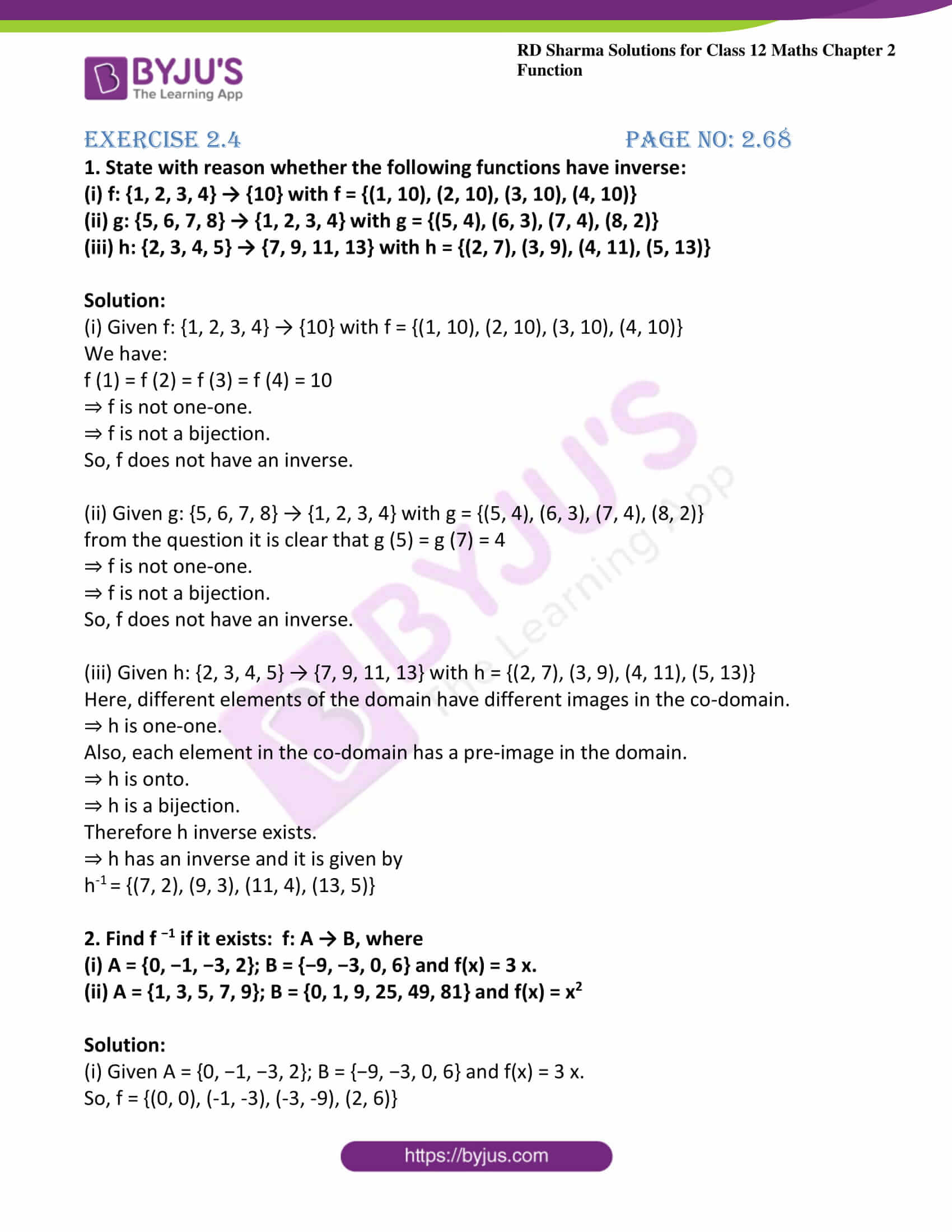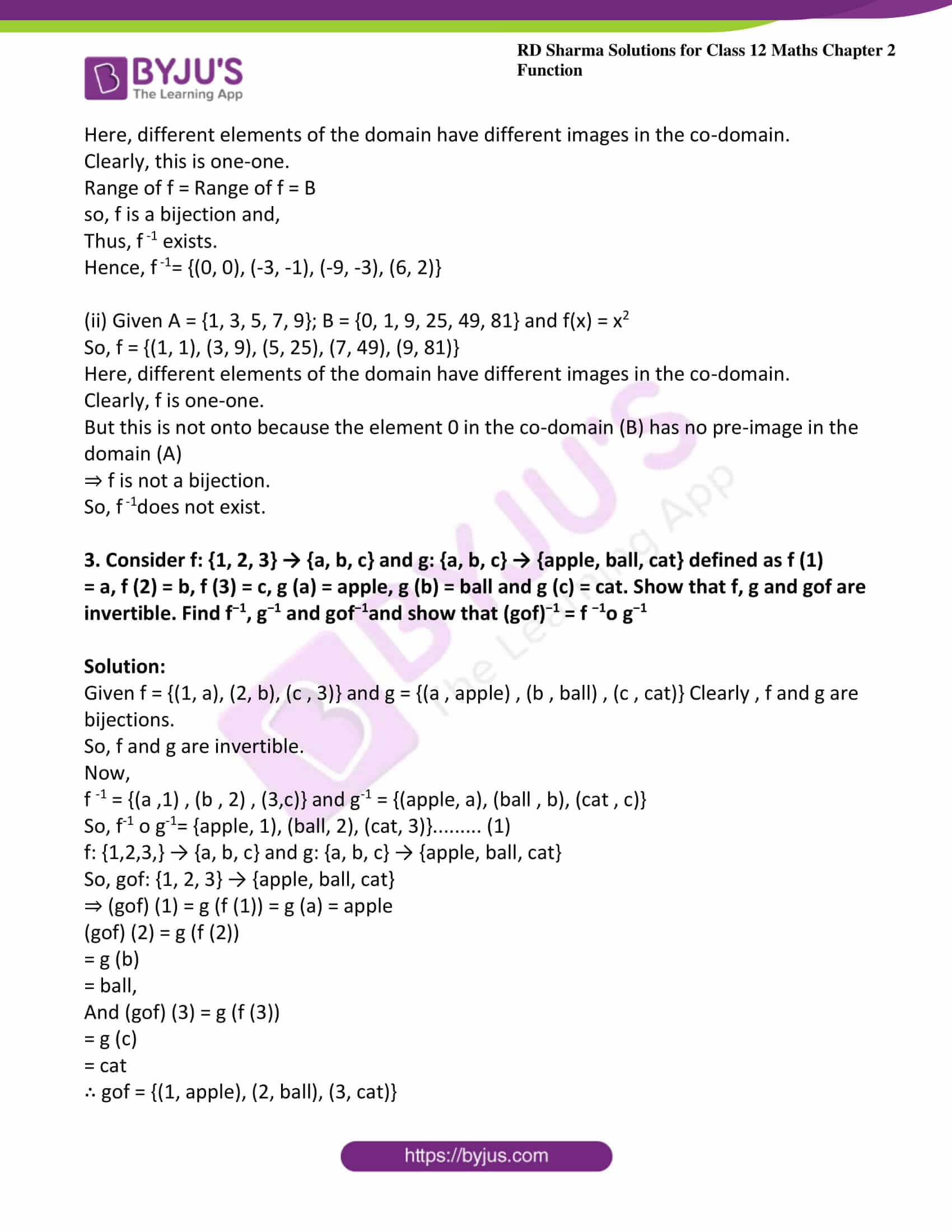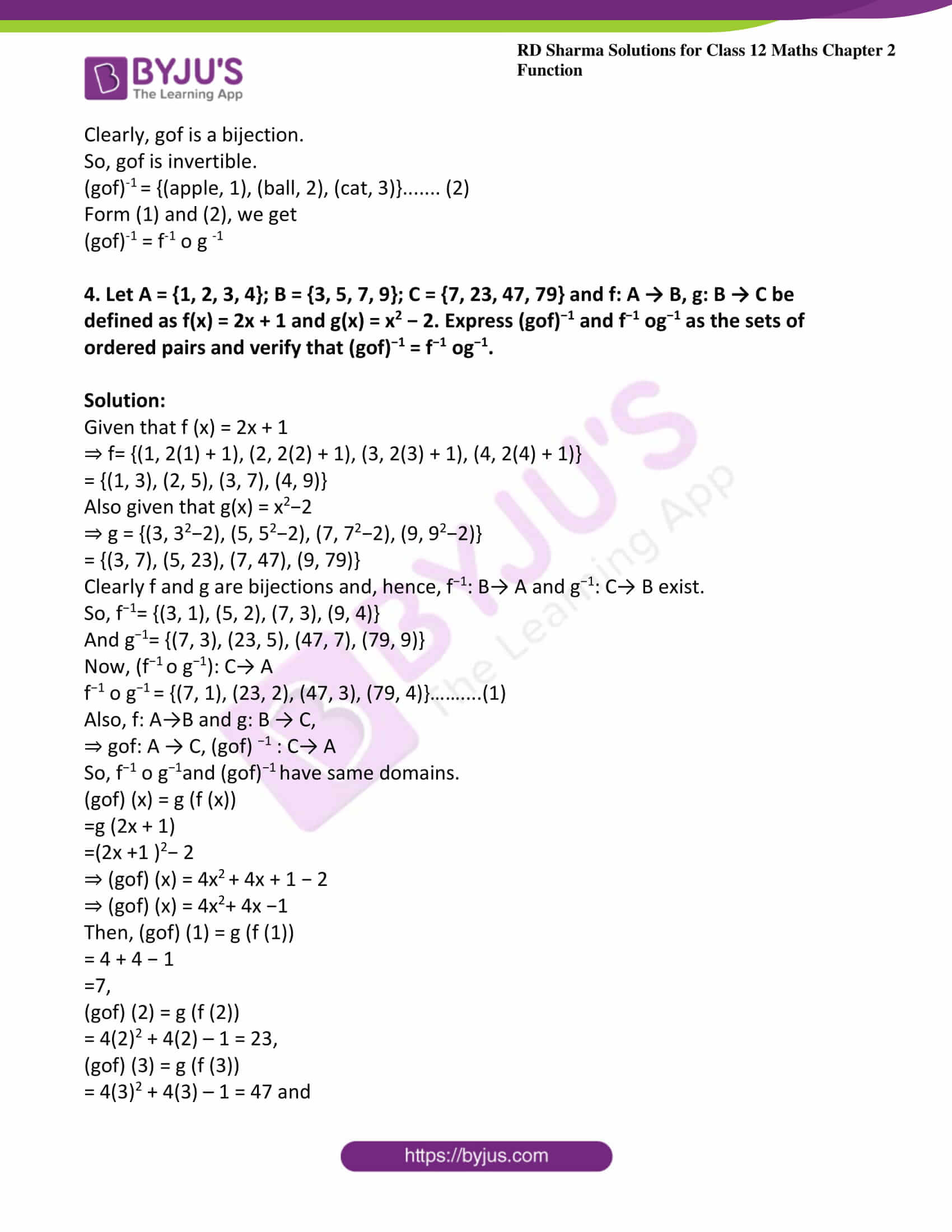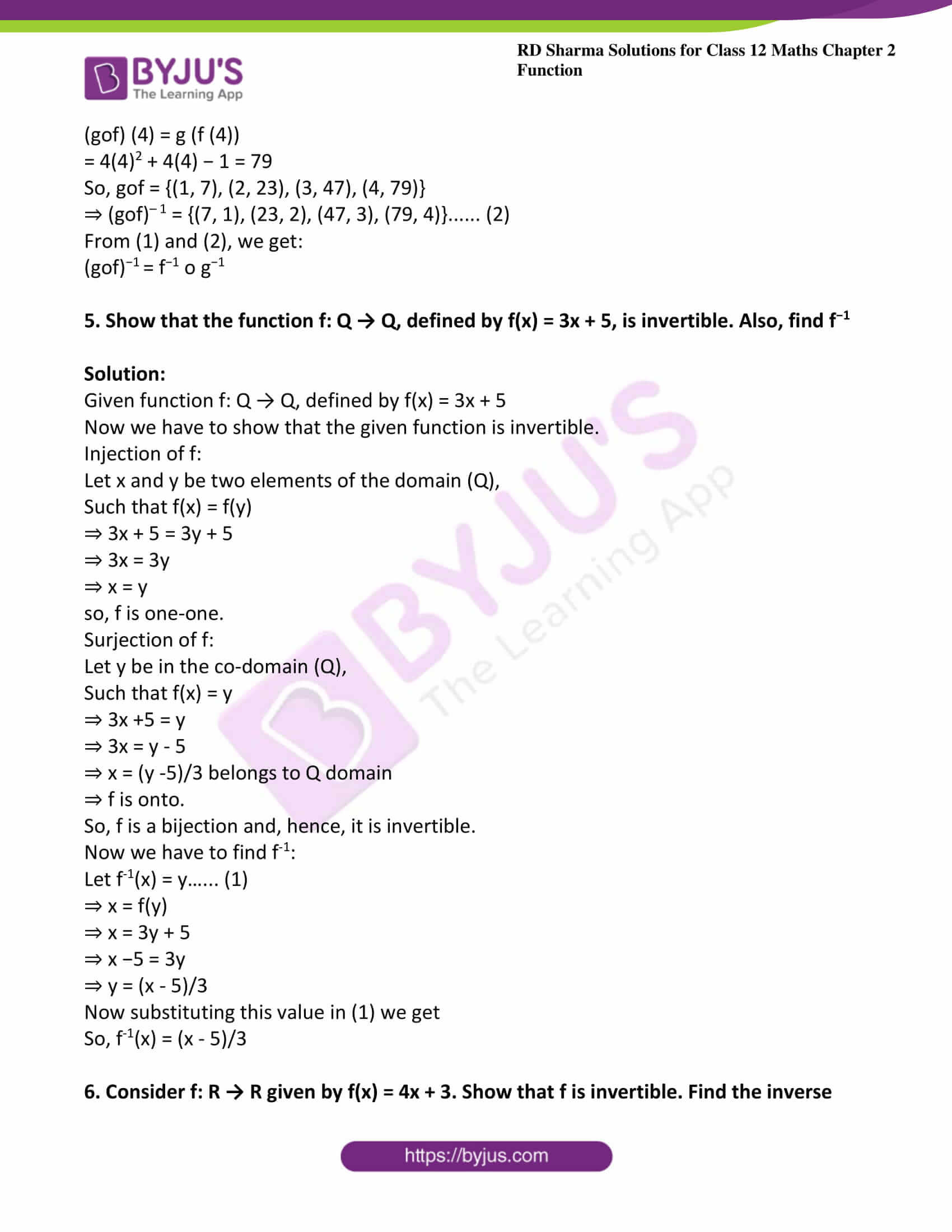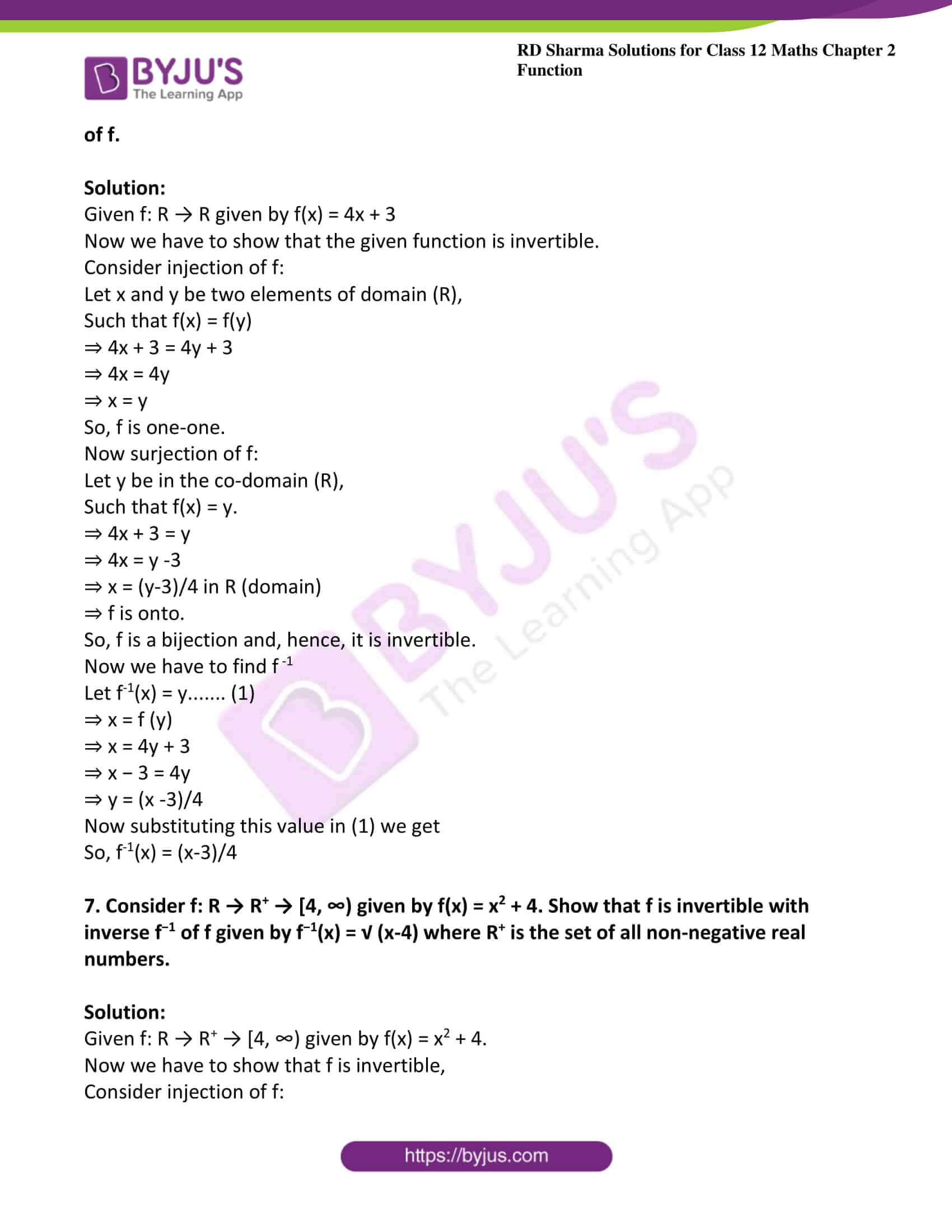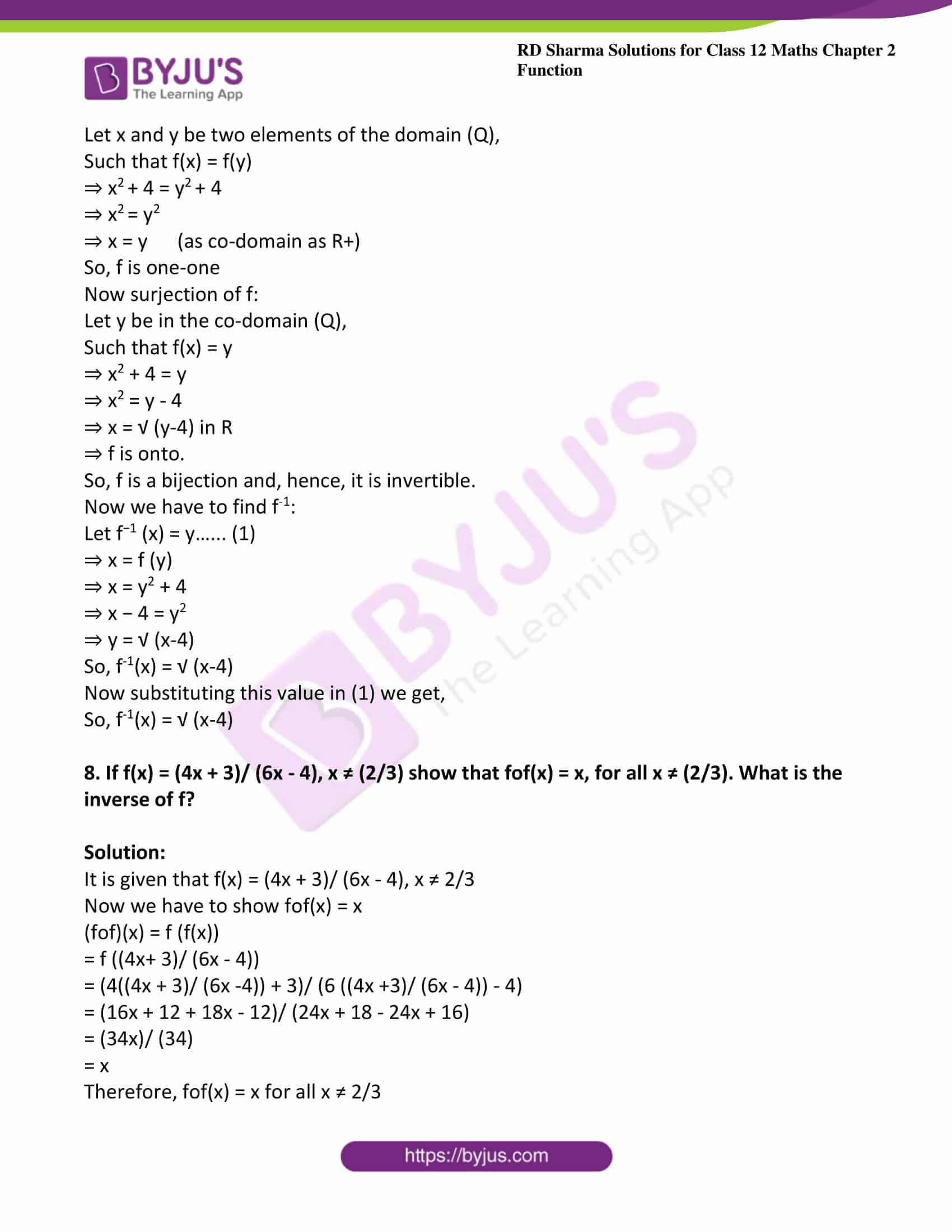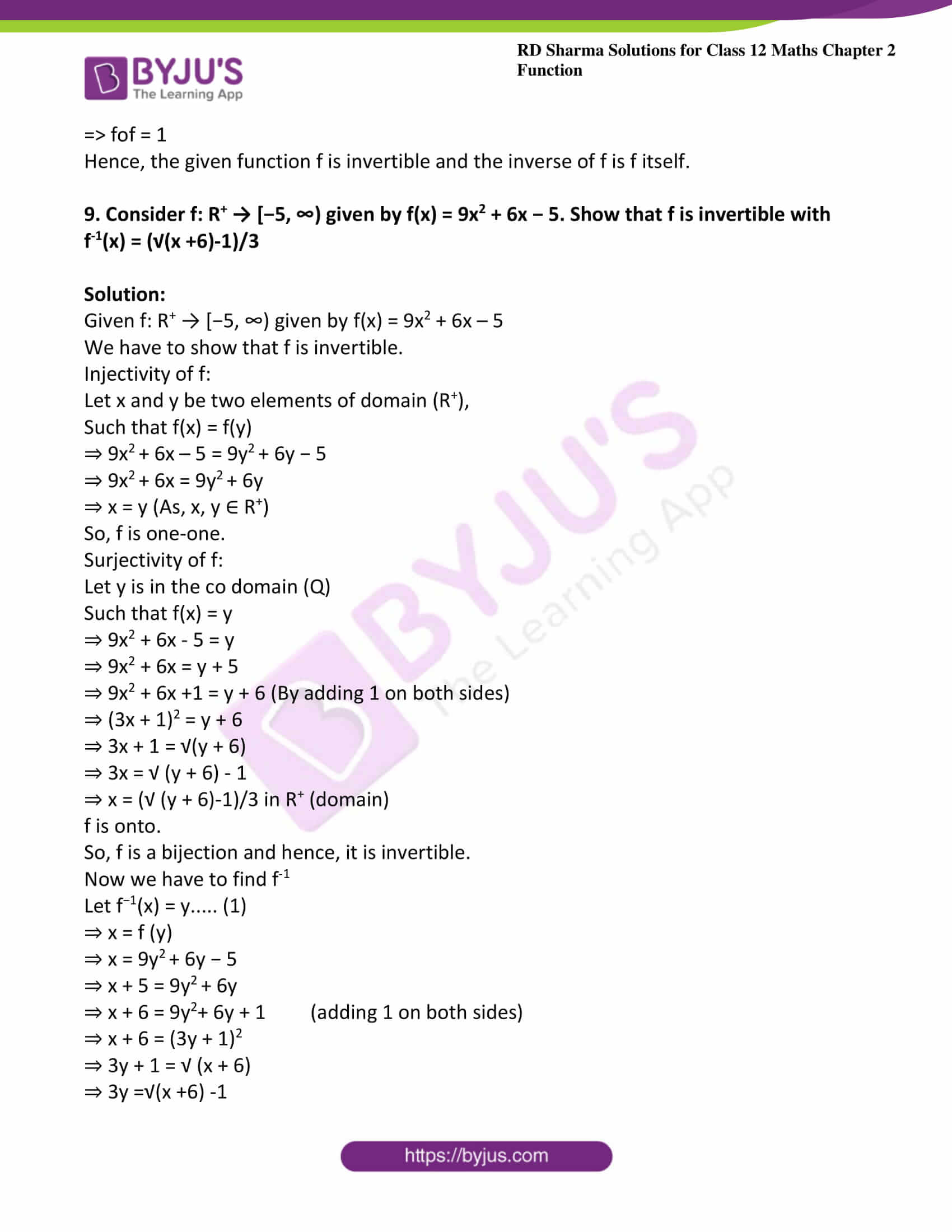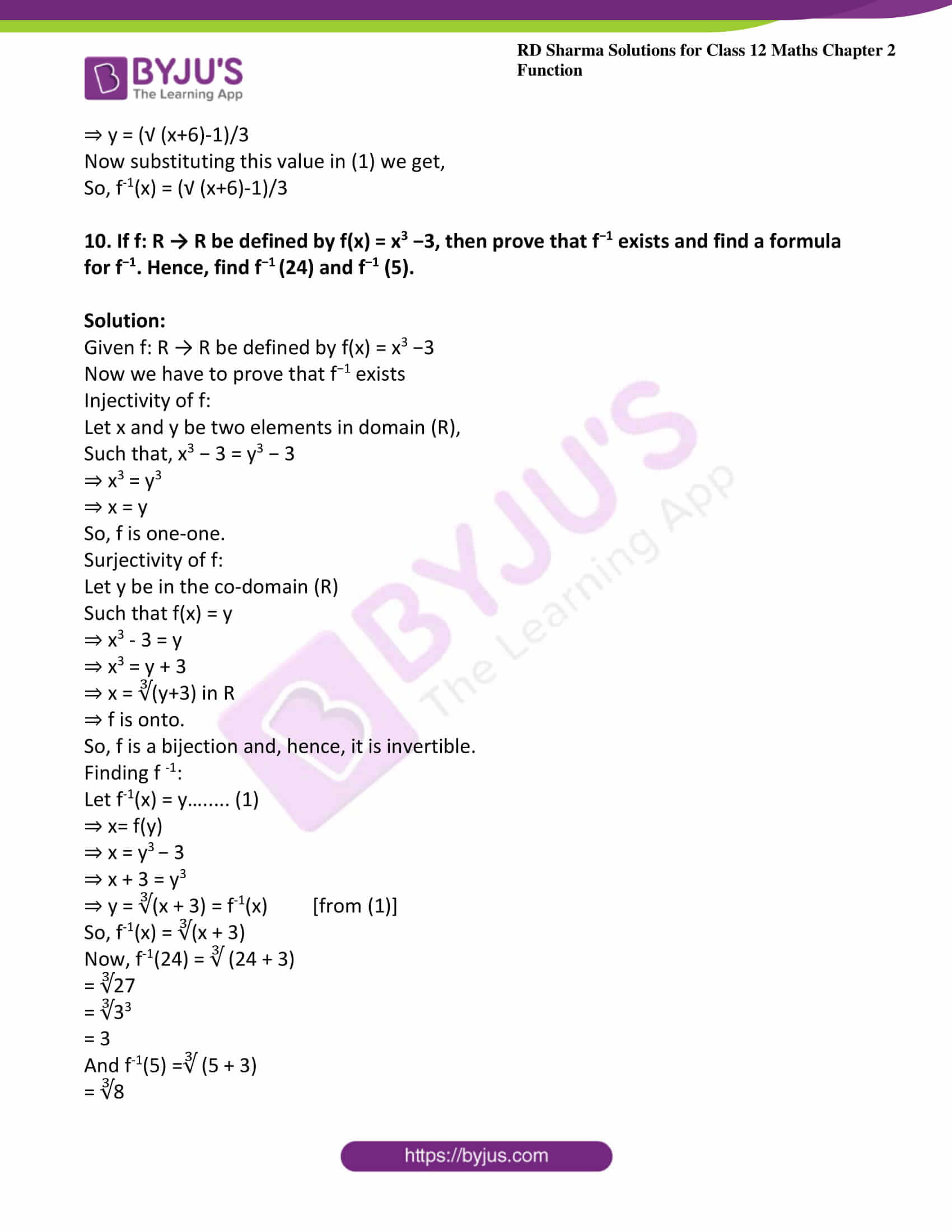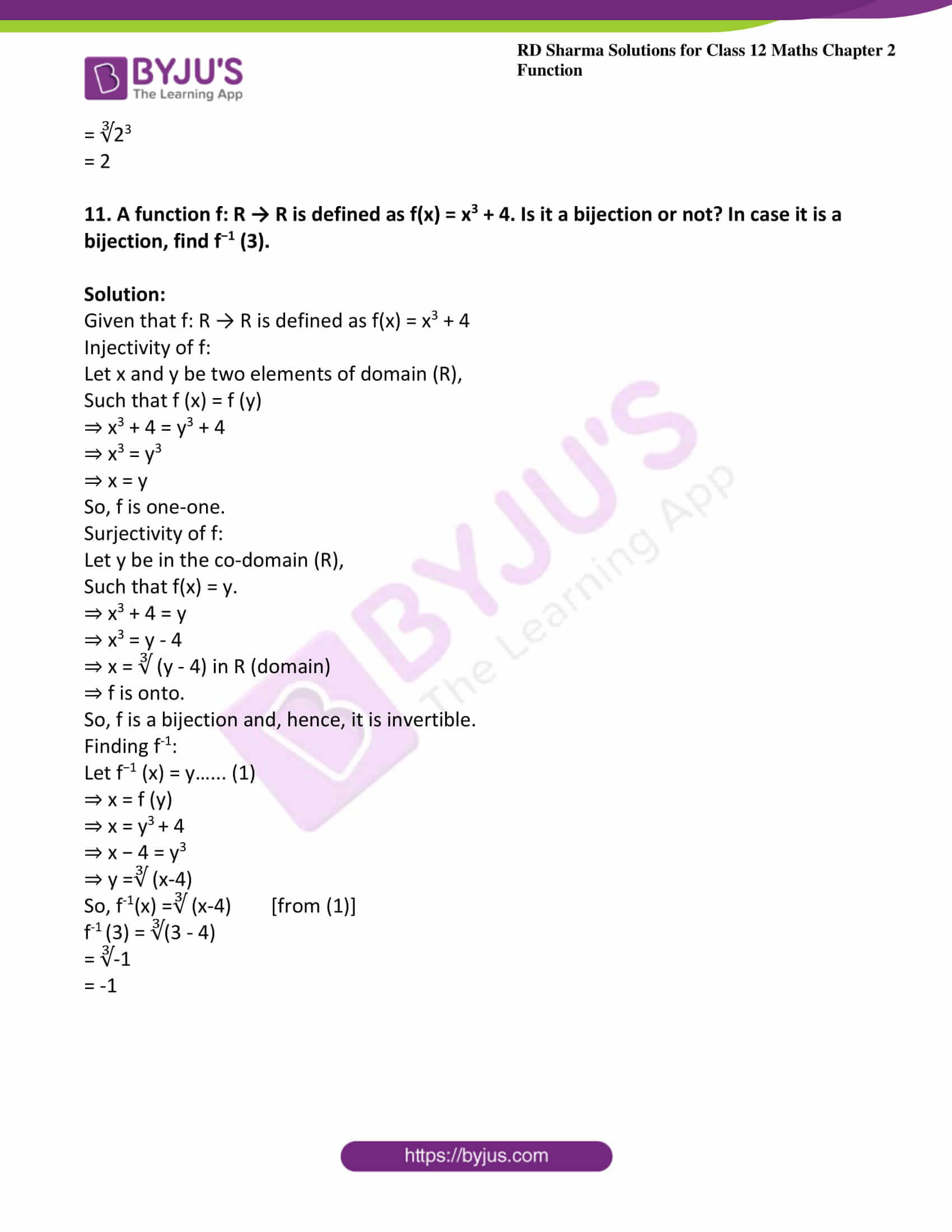### Access other exercises of RD Sharma Solutions For Class 12 Chapter 2 – Function

Exercise 2.1 Solutions

Exercise 2.2 Solutions

Exercise 2.3 Solutions

### Exercise 2.4 Page No: 2.68

1. State with reason whether the following functions have inverse:
(i) f: {1, 2, 3, 4} → {10} with f = {(1, 10), (2, 10), (3, 10), (4, 10)}

(ii) g: {5, 6, 7, 8} → {1, 2, 3, 4} with g = {(5, 4), (6, 3), (7, 4), (8, 2)}

(iii) h: {2, 3, 4, 5} → {7, 9, 11, 13} with h = {(2, 7), (3, 9), (4, 11), (5, 13)}

Solution:

(i) Given f: {1, 2, 3, 4} → {10} with f = {(1, 10), (2, 10), (3, 10), (4, 10)}

We have:

f (1) = f (2) = f (3) = f (4) = 10

⇒ f is not one-one.

⇒ f is not a bijection.

So, f does not have an inverse.

(ii) Given g: {5, 6, 7, 8} → {1, 2, 3, 4} with g = {(5, 4), (6, 3), (7, 4), (8, 2)}

from the question it is clear that g (5) = g (7) = 4

⇒ f is not one-one.

⇒ f is not a bijection.

So, f does not have an inverse.

(iii) Given h: {2, 3, 4, 5} → {7, 9, 11, 13} with h = {(2, 7), (3, 9), (4, 11), (5, 13)}

Here, different elements of the domain have different images in the co-domain.

⇒ h is one-one.

Also, each element in the co-domain has a pre-image in the domain.

⇒ h is onto.

⇒ h is a bijection.

Therefore h inverse exists.

⇒ h has an inverse and it is given by

h-1 = {(7, 2), (9, 3), (11, 4), (13, 5)}

2. Find f −1 if it exists:  f: A → B, where

(i) A = {0, −1, −3, 2}; B = {−9, −3, 0, 6} and f(x) = 3 x.

(ii) A = {1, 3, 5, 7, 9}; B = {0, 1, 9, 25, 49, 81} and f(x) = x2

Solution:

(i) Given A = {0, −1, −3, 2}; B = {−9, −3, 0, 6} and f(x) = 3 x.

So, f = {(0, 0), (-1, -3), (-3, -9), (2, 6)}

Here, different elements of the domain have different images in the co-domain.

Clearly, this is one-one.

Range of f = Range of f = B

so, f is a bijection and,

Thus, f -1 exists.

Hence, f -1= {(0, 0), (-3, -1), (-9, -3), (6, 2)}

(ii) Given A = {1, 3, 5, 7, 9}; B = {0, 1, 9, 25, 49, 81} and f(x) = x2

So, f = {(1, 1), (3, 9), (5, 25), (7, 49), (9, 81)}

Here, different elements of the domain have different images in the co-domain.

Clearly, f is one-one.

But this is not onto because the element 0 in the co-domain (B) has no pre-image in the domain (A)

⇒ f is not a bijection.

So, f -1does not exist.

3. Consider f: {1, 2, 3} → {a, b, c} and g: {a, b, c} → {apple, ball, cat} defined as f (1) = a, f (2) = b, f (3) = c, g (a) = apple, g (b) = ball and g (c) = cat. Show that f, g and gof are invertible. Find f−1, g−1 and gof−1and show that (gof)−1 = f −1o g−1

Solution:

Given f = {(1, a), (2, b), (c , 3)} and g = {(a , apple) , (b , ball) , (c , cat)} Clearly , f and g are bijections.

So, f and g are invertible.

Now,

-1 = {(a ,1) , (b , 2) , (3,c)} and g-1 = {(apple, a), (ball , b), (cat , c)}

So, f-1 o g-1= {apple, 1), (ball, 2), (cat, 3)}……… (1)

f: {1,2,3,} → {a, b, c} and g: {a, b, c} → {apple, ball, cat}

So, gof: {1, 2, 3} → {apple, ball, cat}

⇒ (gof) (1) = g (f (1)) = g (a) = apple

(gof) (2) = g (f (2))

= g (b)

= ball,

And (gof) (3) = g (f (3))

= g (c)

= cat

∴ gof = {(1, apple), (2, ball), (3, cat)}

Clearly, gof is a bijection.

So, gof is invertible.

(gof)-1 = {(apple, 1), (ball, 2), (cat, 3)}……. (2)

Form (1) and (2), we get

(gof)-1 = f-1 o g -1

4. Let A = {1, 2, 3, 4}; B = {3, 5, 7, 9}; C = {7, 23, 47, 79} and f: A → B, g: B → C be defined as f(x) = 2x + 1 and g(x) = x2 − 2. Express (gof)−1 and f−1 og−1 as the sets of ordered pairs and verify that (gof)−1 = f−1 og−1.

Solution:

Given that f (x) = 2x + 1

⇒ f= {(1, 2(1) + 1), (2, 2(2) + 1), (3, 2(3) + 1), (4, 2(4) + 1)}

= {(1, 3), (2, 5), (3, 7), (4, 9)}

Also given that g(x) = x2−2

⇒ g = {(3, 32−2), (5, 52−2), (7, 72−2), (9, 92−2)}

= {(3, 7), (5, 23), (7, 47), (9, 79)}

Clearly f and g are bijections and, hence, f−1: B→ A and g−1: C→ B exist.

So, f−1= {(3, 1), (5, 2), (7, 3), (9, 4)}

And g−1= {(7, 3), (23, 5), (47, 7), (79, 9)}

Now, (f−1 o g−1): C→ A

f−1 o g−1 = {(7, 1), (23, 2), (47, 3), (79, 4)}……….(1)

Also, f: A→B and g: B → C,

⇒ gof: A → C, (gof) −1 : C→ A

So, f−1 o g−1and (gof)−1 have same domains.

(gof) (x) = g (f (x))

=g (2x + 1)

=(2x +1 )2− 2

⇒ (gof) (x) = 4x2 + 4x + 1 − 2

⇒ (gof) (x) = 4x2+ 4x −1

Then, (gof) (1) = g (f (1))

= 4 + 4 − 1

=7,

(gof) (2) = g (f (2))

= 4(2)2 + 4(2) – 1 = 23,

(gof) (3) = g (f (3))

= 4(3)2 + 4(3) – 1 = 47 and

(gof) (4) = g (f (4))

= 4(4)2 + 4(4) − 1 = 79

So, gof = {(1, 7), (2, 23), (3, 47), (4, 79)}

⇒ (gof)– 1 = {(7, 1), (23, 2), (47, 3), (79, 4)}…… (2)

From (1) and (2), we get:

(gof)−1 = f−1 o g−1

5. Show that the function f: Q → Q, defined by f(x) = 3x + 5, is invertible. Also, find f−1

Solution:

Given function f: Q → Q, defined by f(x) = 3x + 5

Now we have to show that the given function is invertible.

Injection of f:

Let x and y be two elements of the domain (Q),

Such that f(x) = f(y)

⇒ 3x + 5 = 3y + 5

⇒ 3x = 3y

⇒ x = y

so, f is one-one.

Surjection of f:

Let y be in the co-domain (Q),

Such that f(x) = y

⇒ 3x +5 = y

⇒ 3x = y – 5

⇒ x = (y -5)/3 belongs to Q domain

⇒ f is onto.

So, f is a bijection and, hence, it is invertible.

Now we have to find f-1:

Let f-1(x) = y…… (1)

⇒ x = f(y)

⇒ x = 3y + 5

⇒ x −5 = 3y

⇒ y = (x – 5)/3

Now substituting this value in (1) we get

So, f-1(x) = (x – 5)/3

6. Consider f: R → R given by f(x) = 4x + 3. Show that f is invertible. Find the inverse of f.

Solution:

Given f: R → R given by f(x) = 4x + 3

Now we have to show that the given function is invertible.

Consider injection of f:

Let x and y be two elements of domain (R),

Such that f(x) = f(y)

⇒ 4x + 3 = 4y + 3

⇒ 4x = 4y

⇒ x = y

So, f is one-one.

Now surjection of f:

Let y be in the co-domain (R),

Such that f(x) = y.

⇒ 4x + 3 = y

⇒ 4x = y -3

⇒ x = (y-3)/4 in R (domain)

⇒ f is onto.

So, f is a bijection and, hence, it is invertible.

Now we have to find f -1

Let f-1(x) = y……. (1)

⇒ x = f (y)

⇒ x = 4y + 3

⇒ x − 3 = 4y

⇒ y = (x -3)/4

Now substituting this value in (1) we get

So, f-1(x) = (x-3)/4

7. Consider f: R → R+ → [4, ∞) given by f(x) = x2 + 4. Show that f is invertible with inverse f−1 of f given by f−1(x) = √ (x-4) where R+ is the set of all non-negative real numbers.

Solution:

Given f: R → R+ → [4, ∞) given by f(x) = x2 + 4.

Now we have to show that f is invertible,

Consider injection of f:

Let x and y be two elements of the domain (Q),

Such that f(x) = f(y)

⇒ x2 + 4 = y2 + 4

⇒ x2 = y2

⇒ x = y      (as co-domain as R+)

So, f is one-one

Now surjection of f:

Let y be in the co-domain (Q),

Such that f(x) = y

⇒ x2 + 4 = y

⇒ x2 = y – 4

⇒ x = √ (y-4) in R

⇒ f is onto.

So, f is a bijection and, hence, it is invertible.

Now we have to find f-1:

Let f−1 (x) = y…… (1)

⇒ x = f (y)

⇒ x = y2 + 4

⇒ x − 4 = y2

⇒ y = √ (x-4)

So, f-1(x) = √ (x-4)

Now substituting this value in (1) we get,

So, f-1(x) = √ (x-4)

8. If f(x) = (4x + 3)/ (6x – 4), x ≠ (2/3) show that fof(x) = x, for all x ≠ (2/3). What is the inverse of f?

Solution:

It is given that f(x) = (4x + 3)/ (6x – 4), x ≠ 2/3

Now we have to show fof(x) = x

(fof)(x) = f (f(x))

= f ((4x+ 3)/ (6x – 4))

= (4((4x + 3)/ (6x -4)) + 3)/ (6 ((4x +3)/ (6x – 4)) – 4)

= (16x + 12 + 18x – 12)/ (24x + 18 – 24x + 16)

= (34x)/ (34)

= x

Therefore, fof(x) = x for all x ≠ 2/3

=> fof = 1

Hence, the given function f is invertible and the inverse of f is f itself.

9. Consider f: R+ → [−5, ∞) given by f(x) = 9x2 + 6x − 5. Show that f is invertible with

f-1(x) = (√(x +6)-1)/3

Solution:

Given f: R+ → [−5, ∞) given by f(x) = 9x2 + 6x – 5

We have to show that f is invertible.

Injectivity of f:

Let x and y be two elements of domain (R+),

Such that f(x) = f(y)

⇒ 9x2 + 6x – 5 = 9y2 + 6y − 5

⇒ 9x2 + 6x = 9y2 + 6y

⇒ x = y (As, x, y ∈ R+)

So, f is one-one.

Surjectivity of f:

Let y is in the co domain (Q)

Such that f(x) = y

⇒ 9x2 + 6x – 5 = y

⇒ 9x2 + 6x = y + 5

⇒ 9x2 + 6x +1 = y + 6 (By adding 1 on both sides)

⇒ (3x + 1)2 = y + 6

⇒ 3x + 1 = √(y + 6)

⇒ 3x = √ (y + 6) – 1

⇒ x = (√ (y + 6)-1)/3 in R+ (domain)

f is onto.

So, f is a bijection and hence, it is invertible.

Now we have to find f-1

Let f−1(x) = y….. (1)

⇒ x = f (y)

⇒ x = 9y2 + 6y − 5

⇒ x + 5 = 9y2 + 6y

⇒ x + 6 = 9y2+ 6y + 1         (adding 1 on both sides)

⇒ x + 6 = (3y + 1)2

⇒ 3y + 1 = √ (x + 6)

⇒ 3y =√(x +6) -1

⇒ y = (√ (x+6)-1)/3

Now substituting this value in (1) we get,

So, f-1(x) = (√ (x+6)-1)/3

10. If f: R → R be defined by f(x) = x3 −3, then prove that f−1 exists and find a formula for f−1. Hence, find f−1 (24) and f−1 (5).

Solution:

Given f: R → R be defined by f(x) = x3 −3

Now we have to prove that f−1 exists

Injectivity of f:

Let x and y be two elements in domain (R),

Such that, x3 − 3 = y3 − 3

⇒ x3 = y3

⇒ x = y

So, f is one-one.

Surjectivity of f:

Let y be in the co-domain (R)

Such that f(x) = y

⇒ x3 – 3 = y

⇒ x3 = y + 3

⇒ x = ∛(y+3) in R

⇒ f is onto.

So, f is a bijection and, hence, it is invertible.

Finding f -1:

Let f-1(x) = y…….. (1)

⇒ x= f(y)

⇒ x = y3 − 3

⇒ x + 3 = y3

⇒ y = ∛(x + 3) = f-1(x)         [from (1)]

So, f-1(x) = ∛(x + 3)

Now, f-1(24) = ∛ (24 + 3)

= ∛27

= ∛33

= 3

And f-1(5) =∛ (5 + 3)

= ∛8

= ∛23

= 2

11. A function f: R → R is defined as f(x) = x3 + 4. Is it a bijection or not? In case it is a bijection, find f−1 (3).

Solution:

Given that f: R → R is defined as f(x) = x3 + 4

Injectivity of f:

Let x and y be two elements of domain (R),

Such that f (x) = f (y)

⇒ x3 + 4 = y3 + 4

⇒ x3 = y3

⇒ x = y

So, f is one-one.

Surjectivity of f:

Let y be in the co-domain (R),

Such that f(x) = y.

⇒ x3 + 4 = y

⇒ x3 = y – 4

⇒ x = ∛ (y – 4) in R (domain)

⇒ f is onto.

So, f is a bijection and, hence, it is invertible.

Finding f-1:

Let f−1 (x) = y…… (1)

⇒ x = f (y)

⇒ x = y3 + 4

⇒ x − 4 = y3

⇒ y =∛ (x-4)

So, f-1(x) =∛ (x-4)        [from (1)]

f-1 (3) = ∛(3 – 4)

= ∛-1

= -1